Examples

Chapter 3 Class 11 Trigonometric Functions
Serial order wise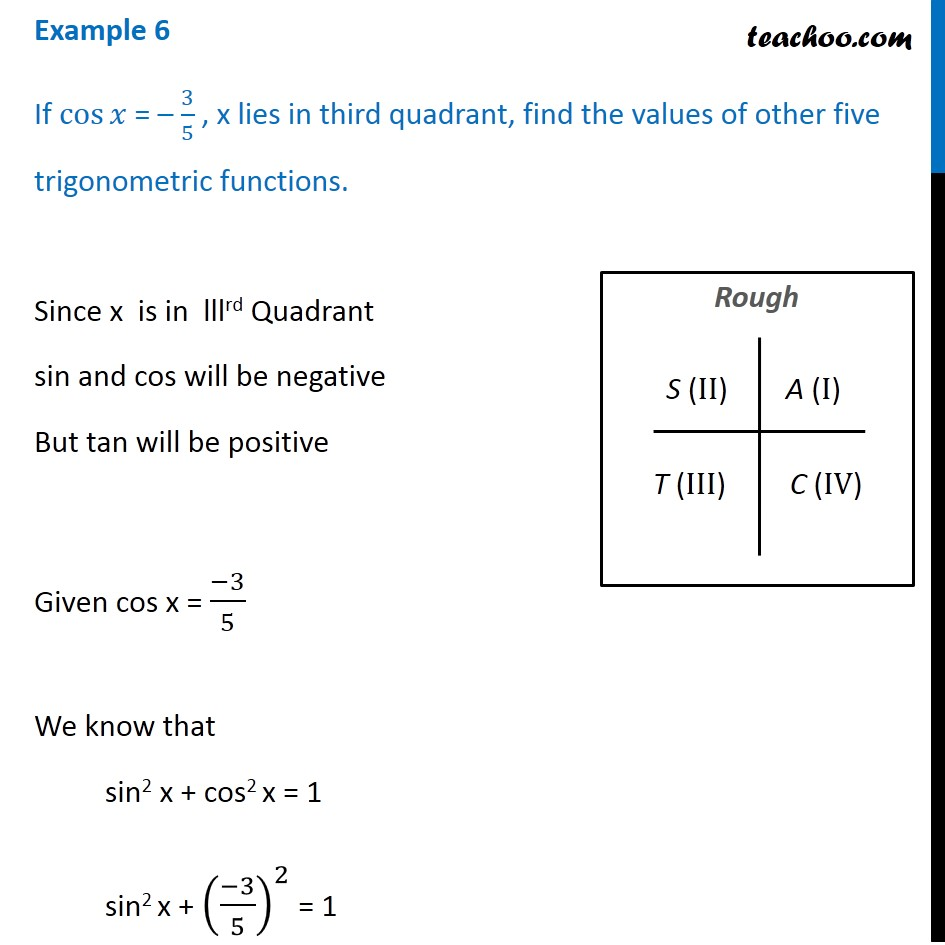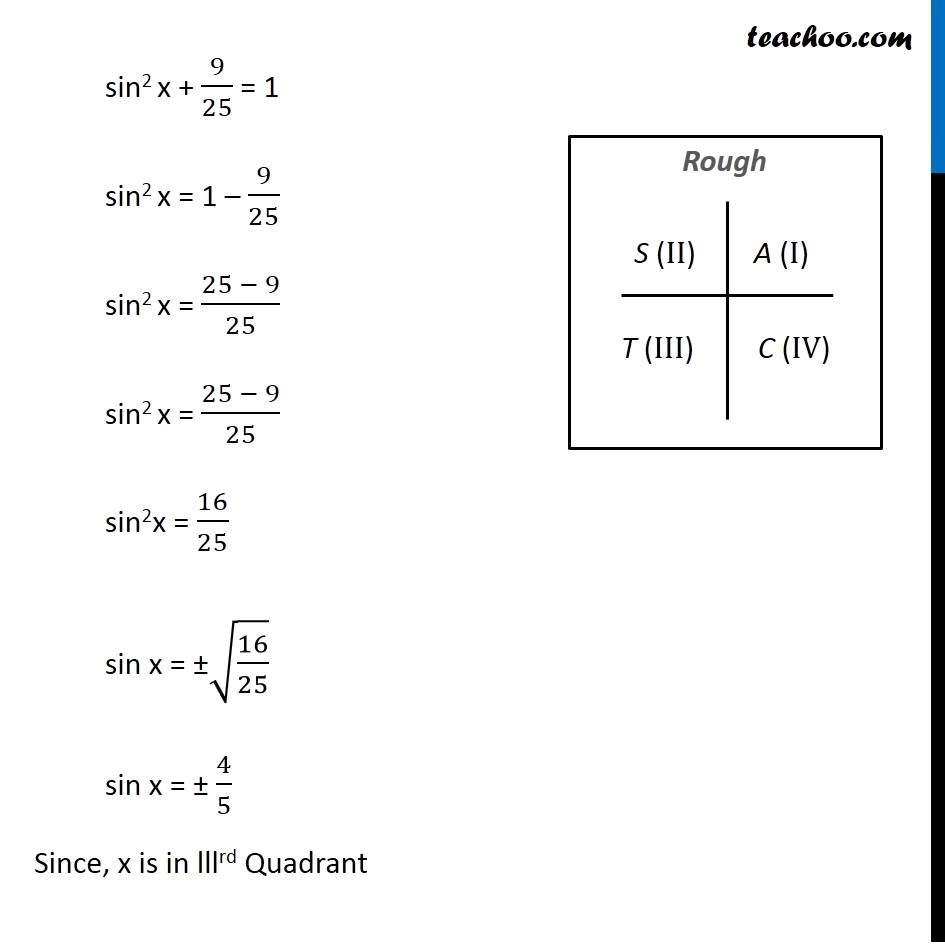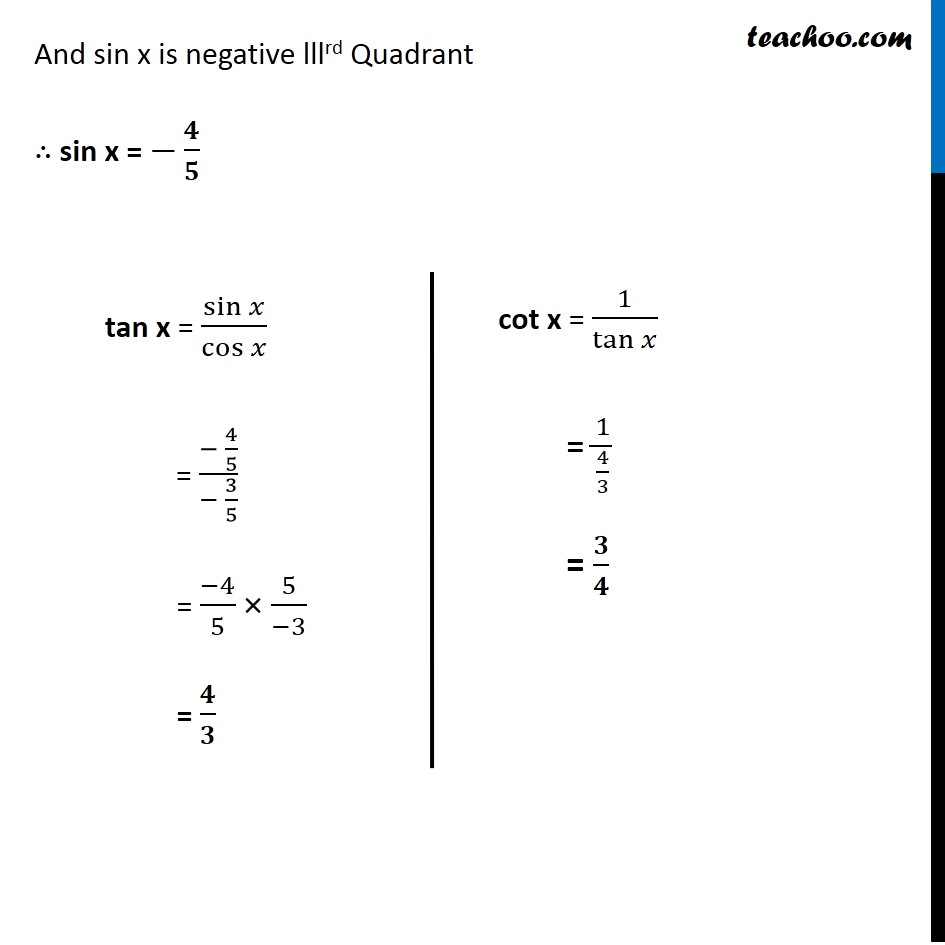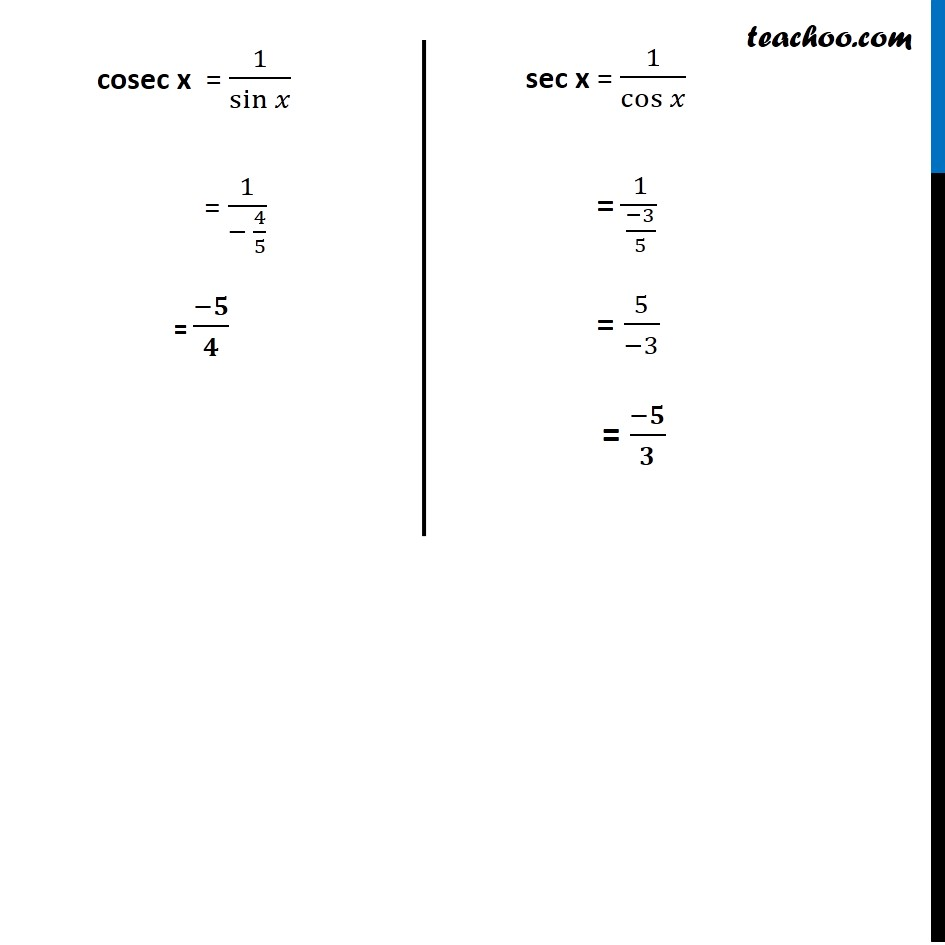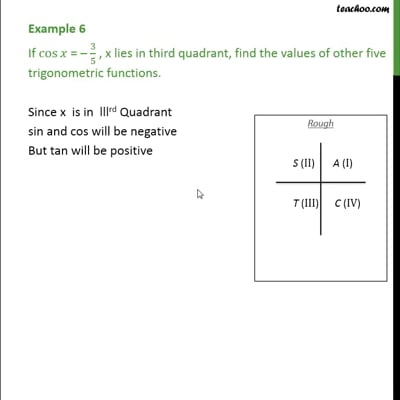This video is only available for Teachoo black users

Maths Crash Course - Live lectures + all videos + Real time Doubt solving!

### Transcript

Example 6 If cos⁡𝑥 = – 3/5 , x lies in third quadrant, find the values of other five trigonometric functions. Since x is in lllrd Quadrant sin and cos will be negative But tan will be positive Given cos x = (−3)/5 We know that sin2 x + cos2 x = 1 sin2 x + ((−3)/5)^2 = 1 sin2 x + 9/25 = 1 sin2 x = 1 – 9/25 sin2 x = (25 − 9)/25 sin2 x = (25 − 9)/25 sin2x = 16/25 sin x = ±√(16/25) sin x = ± 4/5 Since, x is in lllrd Quadrant And sin x is negative lllrd Quadrant ∴ sin x = −𝟒/𝟓 tan x = sin⁡𝑥/cos⁡𝑥 = (− 4/5)/(− 3/5) = (−4)/5 × 5/(−3) = 𝟒/𝟑 cot x = 1/tan⁡𝑥 = 1/(4/3) = 𝟑/𝟒 cosec x = 1/sin⁡𝑥 = 1/(− 4/5) = (−𝟓)/𝟒 sec x = 1/cos⁡𝑥 = 1/((−3)/5) = 5/(−3) = (−𝟓)/𝟑